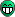# How to attachInterrupt in my code

my code of rfid :
int buffer;
int *buffer_at;
int *buffer_end = buffer + sizeof(buffer);

String checksum;
boolean tagfound = false;

void setup()
{
Serial.begin(9600);

Serial1.begin(9600);
}

void loop()
{

if (Serial1.available()){
delay(20);
buffer_at = buffer;
while ( buffer_at < buffer_end )
{
}
tagfound = true;
Serial1.end();
Serial1.begin(9600);
}

if (tagfound){
buffer_at = buffer;
uint32_t result = 0;
// Skip the preamble
++buffer_at;
// Accumulate the checksum, starting with the first value
int checksum = rfid_get_next();
// We are looking for 4 more values
int i = 4;
while(i–)
{
// Grab the next value
int value = rfid_get_next();
// Add it into the result
result <<= 8;
//Serial.println(result);
result |= value;
//Serial.print(“Xor =”);
//Serial.println(result);
// Xor it into the checksum
checksum ^= value;
}

// Pull out the checksum from the data
int data_checksum = rfid_get_next();

// Print the result
Serial.print(“Tag: “);
Serial.print(result);
if ( checksum == data_checksum )
Serial.println(” OK”);
else
Serial.println(" CHECKSUM FAILED");
// We’re done processing, so there is no current value

tagfound = false;

}

}

int rfid_get_next(void)
{
// sscanf needs a 2-byte space to put the result but we only need one byte.
int hexresult;
// Working space to assemble each byte
static char byte_chars;
// Pull out one byte from this position in the stream
snprintf(byte_chars,3,"%c%c",buffer_at,buffer_at);
sscanf(byte_chars,"%x",&hexresult);
buffer_at += 2;
return hexresult;
}

here i wanna make program where if that tag scan by reader it will call some process, and then if that other tag have been scan it will call other process and that first process will be interrupt.
so hopefully you could help me